# Image Types

Today's post is part of an ongoing (but long delayed) tutorial series on digital image processing using MATLAB. I'm covering topics in roughly the order used in the book Digital Image Processing Using MATLAB.

In the previous post in this series, I discussed the different numeric data types that commonly come into play when doing image processing in MATLAB. Today I want to talk about the four main image types:

• Gray-scale images
• Binary images
• Indexed images
• RGB images

### Contents

#### Gray-Scale Images

A gray-scale image is a matrix whose values represent shades of gray. When the matrix is of type uint8, the integer-valued elements are in the range [0,255]. By convention, the value 0 is displayed as black, and the value 255 is displayed as white. Values in-between are displayed as intermediate shades of gray.

When the matrix is of type uint16, then 0 is displayed as black and 65535 is displayed as white.

For a floating-point matrix, either of type double or single, the value 1.0 is displayed as white.

Originally, the Image Processing Toolbox documentation called these intensity images, and you might still find this term used in some places. Some of our color scientist users complained, though, that the term intensity image meant something slightly different in their field, so we (mostly) changed our terminology.

I = imread('rice.png');
whos I

  Name        Size             Bytes  Class    Attributes

I         256x256            65536  uint8


imshow(I)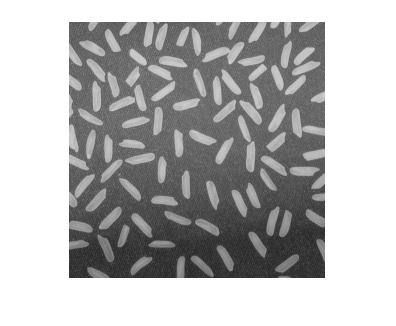#### Binary Images

In image processing, the term binary image refers to a two-valued image whose pixes are either black or white. (Or, a bit more generally, the pixels are either background or foreground.) In MATLAB and the Image Processing Toolbox, we have adopted the convention that binary images are represented as logical matrices. Here's an example of constructing a matrix whose type is logical and then displaying the result as a binary (black-and-white) image.

[xx,yy] = meshgrid(-100:100);
bw = hypot(xx,yy) <= 50;
whos bw

  Name        Size             Bytes  Class      Attributes

bw        201x201            40401  logical


imshow(bw)
title('Binary image')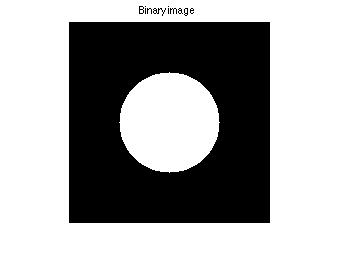Note that a common mistake is to create a uint8 matrix and fill it with 0s and 1s, thinking that it will display as black and white.

bw = zeros(30,30,'uint8');
bw(10:20,10:20) = 1;
imshow(bw,'InitialMagnification','fit')
title('Help, my image is all black!')When a matrix is of type uint8, then the value 1 is not white; it's almost completely black!

#### Indexed Images

An indexed image has two components: an index matrix of integers, commonly called X, and a color map matrix, commonly called map. The matrix map is an M-by-3 matrix of floating-point values (either double or single) in the range [0.0,1.0]. Each row of map specifies the red, green, and blue components of a single color. An indexed image is displayed by mapping values in the index matrix to colors in the color map. A quirk of MATLAB is that this mapping is data-type specific. If the index matrix is floating-point, then the value 1.0 corresponds to the first color in the color map. But if the index matrix is uint8 or uint16, then the value 0 corresponds to the first color. (Maybe I'll explain the reasons for that on another day.)

You display an indexed image by passing both the index matrix and the color map matrix to imshow, like this:

[X,map] = imread('trees.tif');
whos X map

  Name        Size             Bytes  Class     Attributes

X         258x350            90300  uint8
map       256x3               6144  double


imshow(X,map)
title('Indexed image')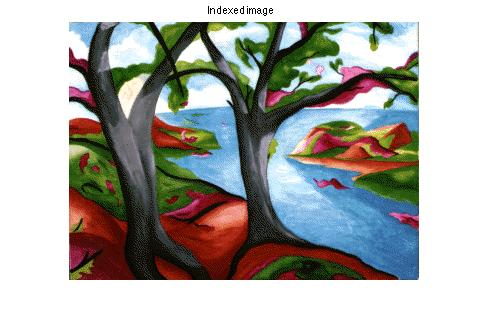#### RGB Images

An RGB image is an M-by-N-by-3 array. For a particular pixel at row r and column c, the three values RGB(r,c,1), RGB(r,c,2), and RGB(r,c,3) specify the red, green, and blue color components of that pixel. A pixel whose color components are [0,0,0] is displayed as black. For a floating-point array, a pixel whose color components are [1.0,1.0,1.0] is displayed as white. For a uint8 or uint16 array, either [255,255,255] or [65535,65535,65535] is displayed as white.

RGB = imread('peppers.png');
whos RGB

  Name        Size                Bytes  Class    Attributes

RGB       384x512x3            589824  uint8


imshow(RGB)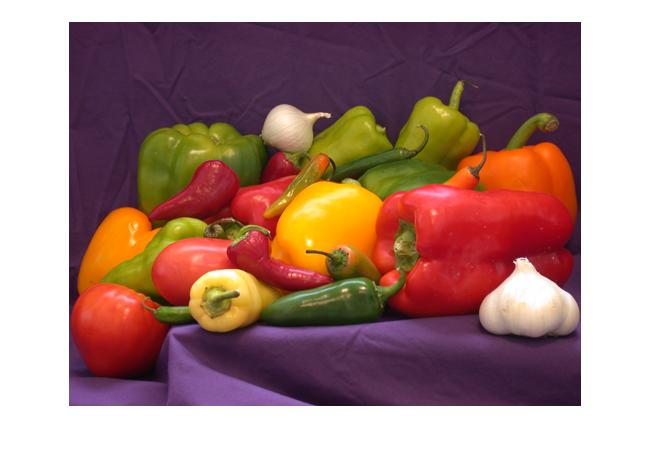You can think of an RGB image as a "stack" of three gray-scale images. These gray-scale images are commonly called the component images.

imshow(RGB(:,:,1))
title('Red component image')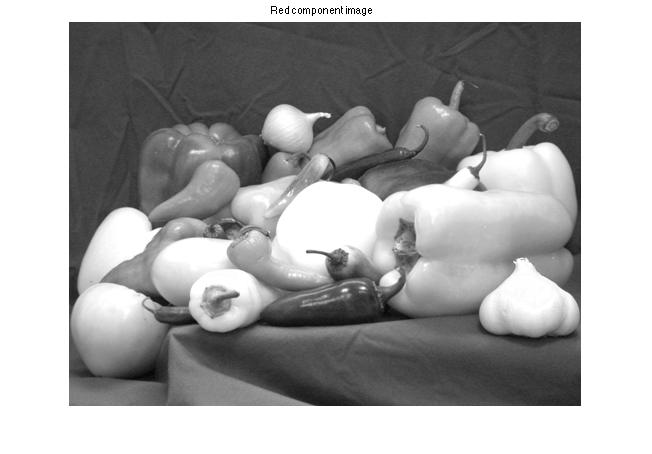imshow(RGB(:,:,2))
title('Green component image')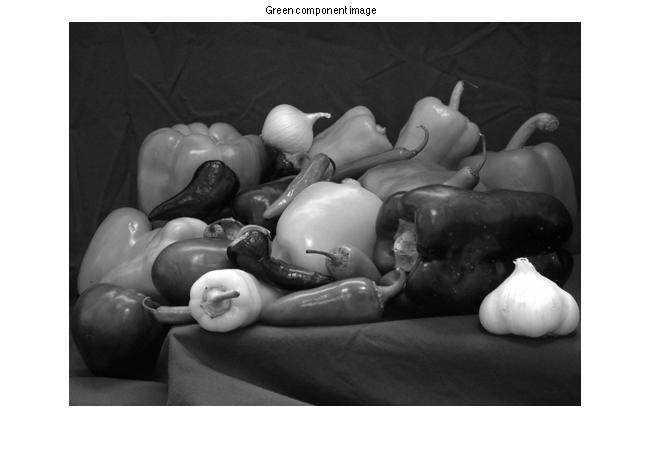imshow(RGB(:,:,3))
title('Blue component image')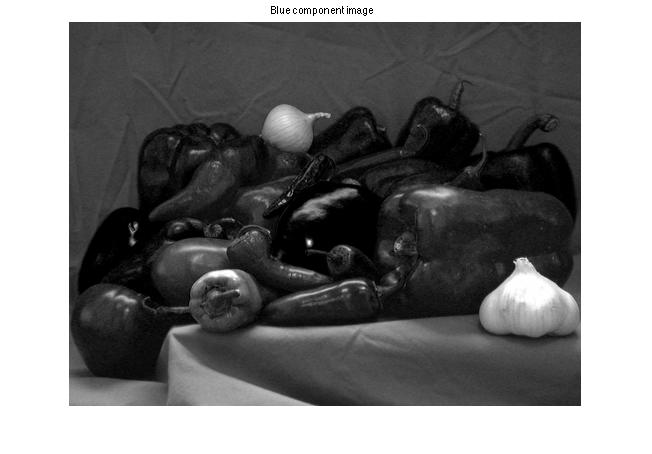In my first year of blogging (2006), I wrote a series of blog posts explaining in great detail how the MATLAB image display model works for various image and data types. The series is still up-to-date and worth reading today. Or, to see it all in one place, take a look at my MATLAB Digest article, "How MATLAB Represents Pixel Colors."

For more information, see Sections 2.7 and 7.1 of Digital Image Processing Using MATLAB.Published with MATLAB® R2014a

|